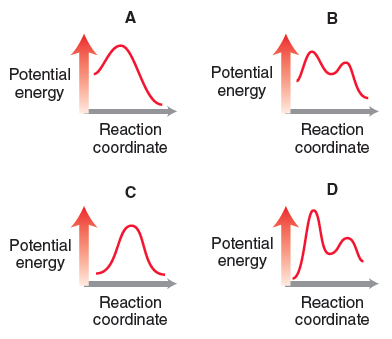# Problem: Consider the following four energy diagrams:Which process will have a value of K eq that is roughly equal to 1?

🤓 Based on our data, we think this question is relevant for Professor Chatterjee's class at HUNTER.

###### Problem Details

Consider the following four energy diagrams:

Which process will have a value of K eq that is roughly equal to 1?What scientific concept do you need to know in order to solve this problem?

Our tutors have indicated that to solve this problem you will need to apply the Chemical Equilibrium concept. If you need more Chemical Equilibrium practice, you can also practice Chemical Equilibrium practice problems.

What is the difficulty of this problem?

Our tutors rated the difficulty ofConsider the following four energy diagrams:Which process wi...as high difficulty.

What professor is this problem relevant for?

Based on our data, we think this problem is relevant for Professor Chatterjee's class at HUNTER.

What textbook is this problem found in?

Our data indicates that this problem or a close variation was asked in Organic Chemistry - Klein 1st Edition. You can also practice Organic Chemistry - Klein 1st Edition practice problems.